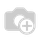# Mimick One2One Implementation

Hi community,

Thank you in advance for any comments / suggestions that could help my problem.

I have two objects currently that I want to uniquely link together. A "container object" and a "calculations" object. I want every container to have one (and only) one calculations object, and one calculations object to have one and only one container object.

I know that one2one is depreciated, but my programming need does not call for inheritance. The two objects have absolutely nothing to do with each other.

Thus, I have tried adding a many2one field in container AND a many2one field in calculations. This has actually worked rather well, however I'm stuck at the final part:

A container object is always created first. So, when I create a container, I can open the "calculations" field from the form, and select "Create new" from the dropdown. My question is this:

When the new form opens to create a "calculations" object, how can I reference the ID of my "container" object, so that I can automatically assign THAT container to my calculations object, by default. In this way, if I don't present the user with a field to set the "container", the default will always be used and thus mimicking a one2one relationship with 2 many2ones.

Does anyone have a suggestion?What you need to set a `context` on "Container" form's `calculation_id` field:.

Assuming that "Container" and a many2one `calculation_id` for the Calculation object, and the Calculation object has a many2one `container_id`:

``````<field name="calculation_id" context="{'default_container_id':  active_id}" />
``````

This will tell the Calculation form to by default set the `container_id` field with the Container's Id.